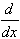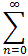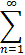#Interactive Real Analysis

Next | Previous | Glossary | Map

## 8.4. Taylor Series

### Example

Find a series for f(x) = 2x/(1-x2)2

This time we need to find a function whose derivative is our function in question, and whose Taylor series we know. Experimenting on scratch paper for a little while will give us, as a guess, the function

g(x) = 1/1-x2

The derivative of this function is g'(x) = 2x/(1-x2)2, our function in question. Thus:

f(x) = g'(x) =g(x) =x2n =x2n =2n x2n-1

for |x| < 1. We have, of course, quietly used substitution for the Geometric series and as a result - voila! - we have a Taylor series centered at zero for our function, just as desired.2n x2n-1 f(x) = 2x/(1-x2)2
Next | Previous | Glossary | Map